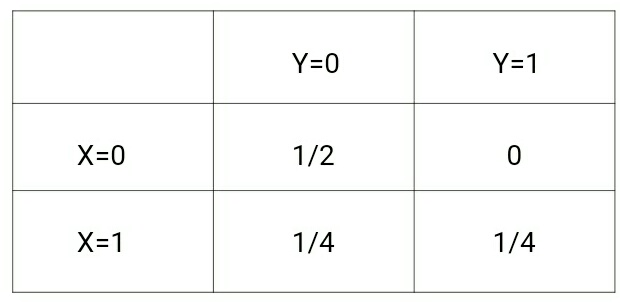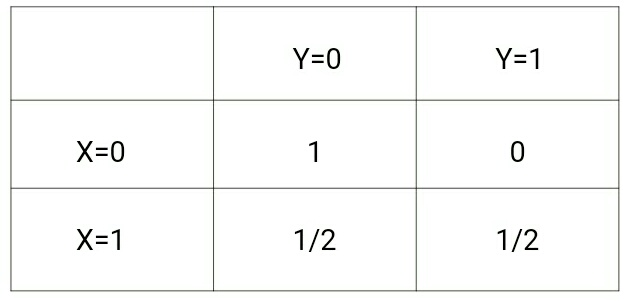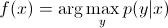×

Search anything:

# Discriminative Model

#### Machine Learning (ML) discriminative modelGet this book -> Problems on Array: For Interviews and Competitive Programming

Discriminative models, also referred to as conditional models, are a class of models used in statistical classification, especially in supervised machine learning.

Unlike the generative modelling, which studies from the joint probability P(x,y), the discriminative modelling studies the P(y|x) i.e, it predicts probability of y(target) when given x(training samples).

• Lets understand this with the help of an mathematical example:

Suppose the input data is x and the set of labels for x is y. Consider following 4 data points:

``````(x,y) –> {(0,0), (0,0), (1,0), (1,1)}
``````

For above data, p(x,y) will be following:while p(y|x) will be following:If we take a look at those two matrices, we will understand the difference between the two probability distributions.

So discriminative algorithms tries to learn p(y|x) directly from the data and then tries to classify data.

On the other hand, generative algorithms tries to learn p(x,y) which can be transformed into p(y|x) later to classify the data. One of the advantages of generative algorithms is that you can use p(x,y) to generate new data similar to existing data. On the other hand, discriminative algorithms generally give better performance in classification tasks.

In discriminative models, to predict the label y from the training example x, we must evaluate:which merely chooses what is the most likely class y considering x. It's like we were trying to model the decision boundary between the classes. This behavior is very clear in neural networks, where the computed weights can be seen as a complexly shaped curve isolating the elements of a class in the space.

# Important Characteristics

• focus on decision boundary.
• more powerful with lot of examples.
• not designed to use unlabeled data.

# Discriminative Classifiers Examples

Discriminative models are preferred in following approches:

• Logistic regression
• Scalar Vector Machine
• Nearest neighbour search
• Conditional Random Fields (CRF)s

# Benefits of Discriminative Model

• Discriminative models is used for getting better accuracy on training data.
• Whenever the training data is big ,the accuracy for future data will be good.
• When the number of parameters is limited, a discriminative model is going to attempt to optimize the prediction of y from x, whereas a generative model will attempt to optimize the joint prediction of x and y. Because of this, discriminative models outperform generative models at conditional prediction tasks.#### Prashant Anand

Bachelor of Technology (2016 to 2020) in Electronics and Communications Engineering at Reva University, Bangalore | Intern at OpenGenus

Discriminative Model